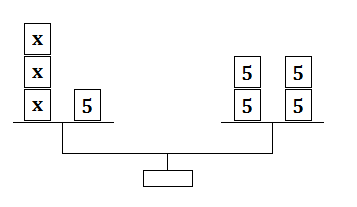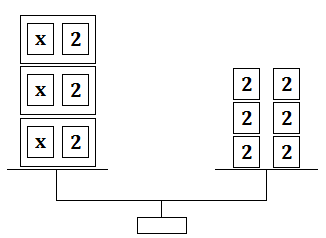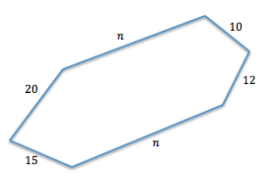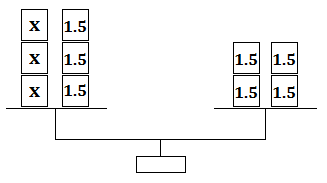# Equations and Inequalities

## Objective

Solve equations in the forms ${px+q=r }$ and ${p(x+q)=r}$ algebraically.

## Common Core Standards

### Core Standards

?

• 7.EE.B.4.A — Solve word problems leading to equations of the form px + q = r and p(x + q) = r, where p, q, and r are specific rational numbers. Solve equations of these forms fluently. Compare an algebraic solution to an arithmetic solution, identifying the sequence of the operations used in each approach. For example, the perimeter of a rectangle is 54 cm. Its length is 6 cm. What is its width?

?

• 6.EE.B.7

## Criteria for Success

?

1. Understand that an equation is a statement of balance, and in solving the equation, the balance must be maintained.
2. Understand solving an equation as a process of undoing operations using inverse operations that adhere to arithmetic properties.
3. Solve equations in the forms $px+q=r$  and $p(x+q)=r$.

## Tips for Teachers

?

In this lesson, students learn the concepts behind the mechanics of solving an equation. In the next two lessons, students will have further opportunities to practice solving equations in contextual situations. Solving equations in these two forms is a fluency expectation in 7th grade. See our Guide to Procedural Skill and Fluency for ideas of activities to use throughout the lesson and remaining part of the unit.

#### Fishtank Plus

• Problem Set
• Student Handout Editor
• Vocabulary Package

## Anchor Problems

?

### Problem 1

A balance is shown below.1. Describe how you can use the balance find the value of $x$.
2. Write an equation to represent the situation shown in the balance.
3. Use the equation to model the actions you did in part A with the balance.
4. Repeat parts A–C using the balance shown below.### Problem 2

Yoshiro has a new puppy. She decides to create an enclosure for her puppy in her backyard. The enclosure is in the shape of a hexagon, with one pair of opposite sides running the same distance along the length of two parallel flowerbeds. A sketch of the enclosure is shown below.If the perimeter of the enclosure is 137 feet, what is the length of each side that runs along the flowerbed? Write an equation to represent the situation, and solve using algebraic properties. Describe each property you use in solving the equation.

#### References

EngageNY Mathematics Grade 7 Mathematics > Module 2 > Topic C > Lesson 22Example 1

Grade 7 Mathematics > Module 2 > Topic C > Lesson 22 of the New York State Common Core Mathematics Curriculum from EngageNY and Great Minds. © 2015 Great Minds. Licensed by EngageNY of the New York State Education Department under the CC BY-NC-SA 3.0 US license. Accessed Dec. 2, 2016, 5:15 p.m..

Modified by The Match Foundation, Inc.

### Problem 3

Solve the equations:

 a.  ${12(x-2)=72}$ b.  ${- {1\over3}x+4=-2}$ c.  ${5.6-2p=13}$

## Problem Set

?The following resources include problems and activities aligned to the objective of the lesson that can be used to create your own problem set.

?

Solve each equation for the variable. Show every step in your work that maintains the balance in each equation.

a.   ${{1\over2}(x+8)=-10}$

b.   ${-5x+12=20}$

c.?